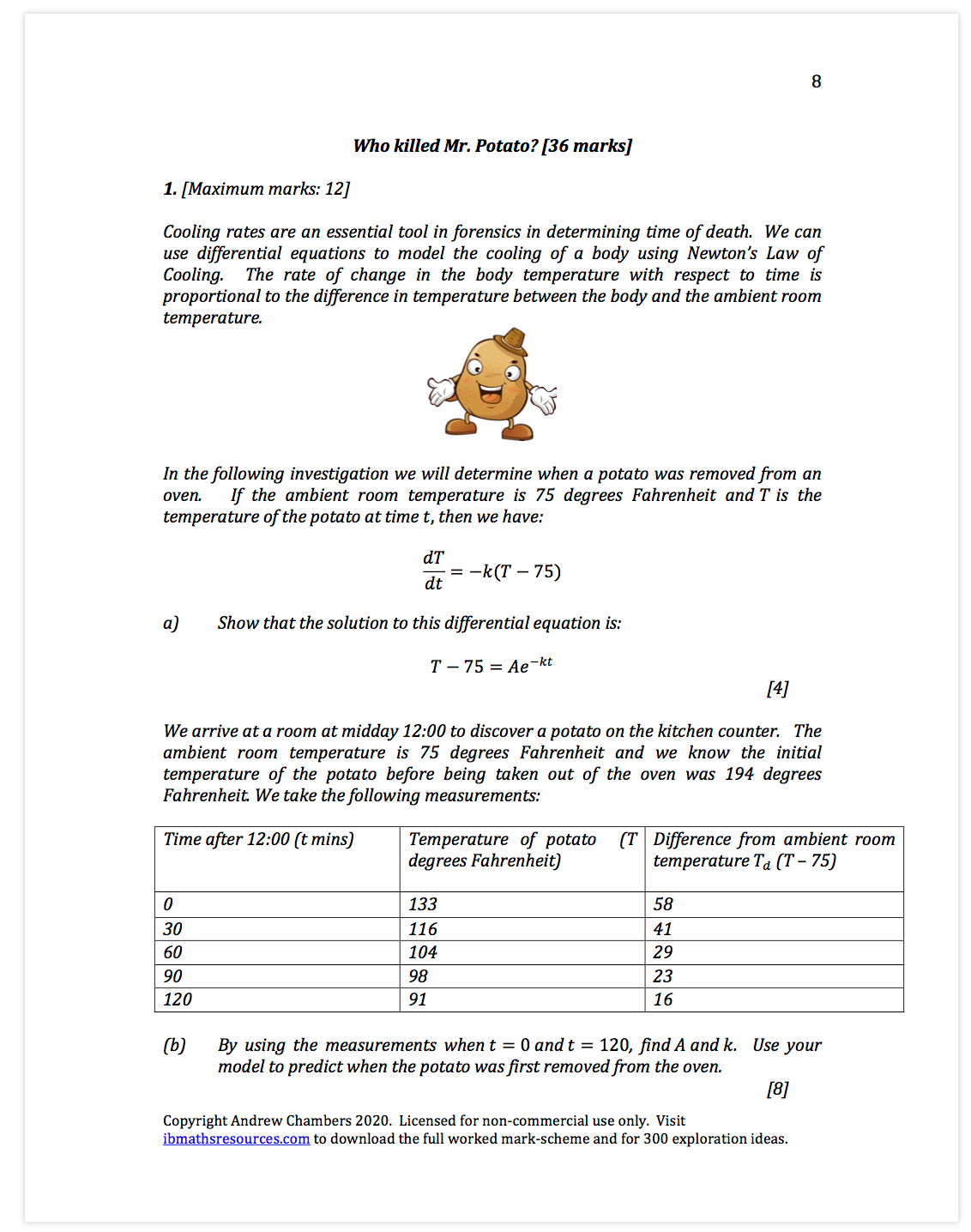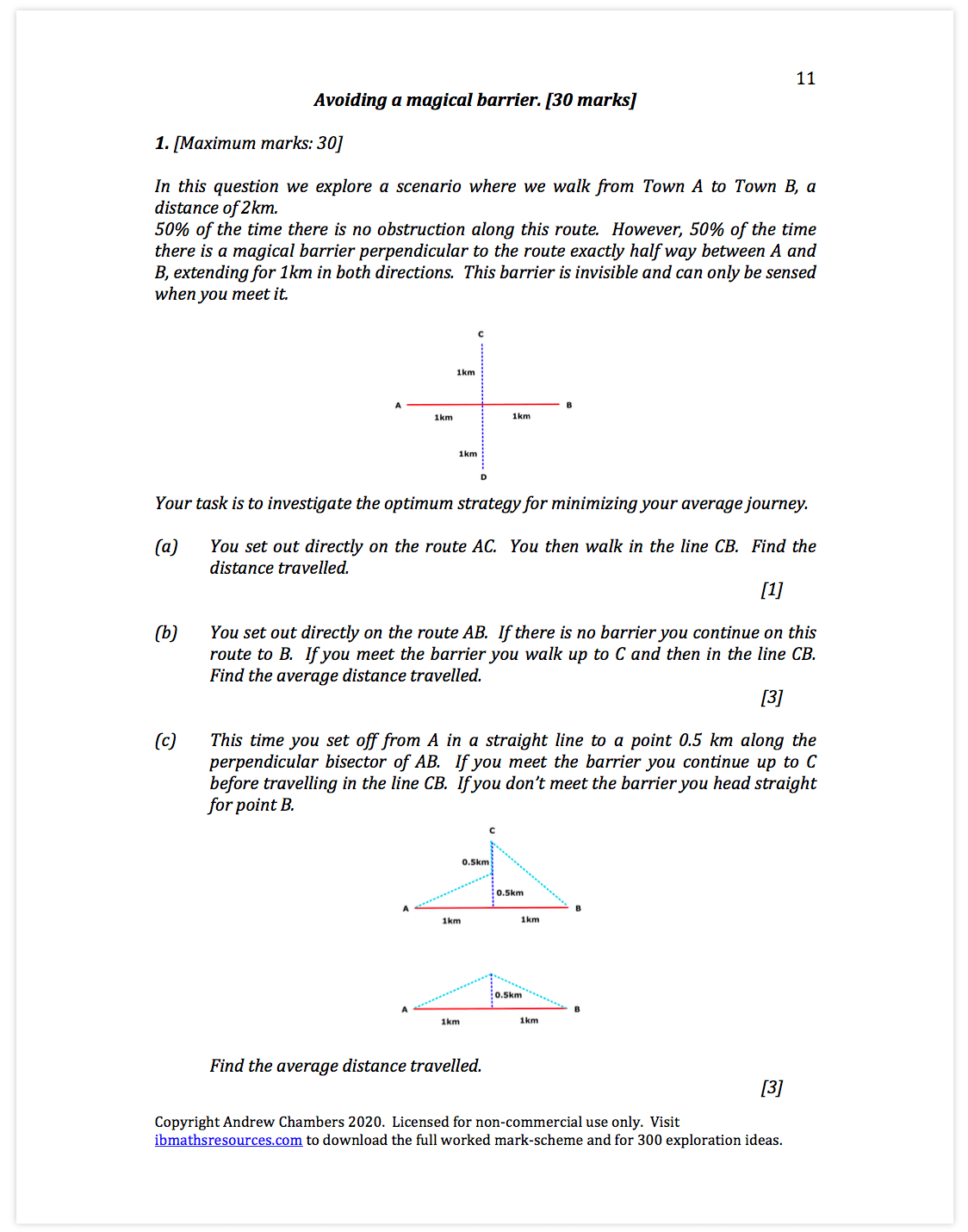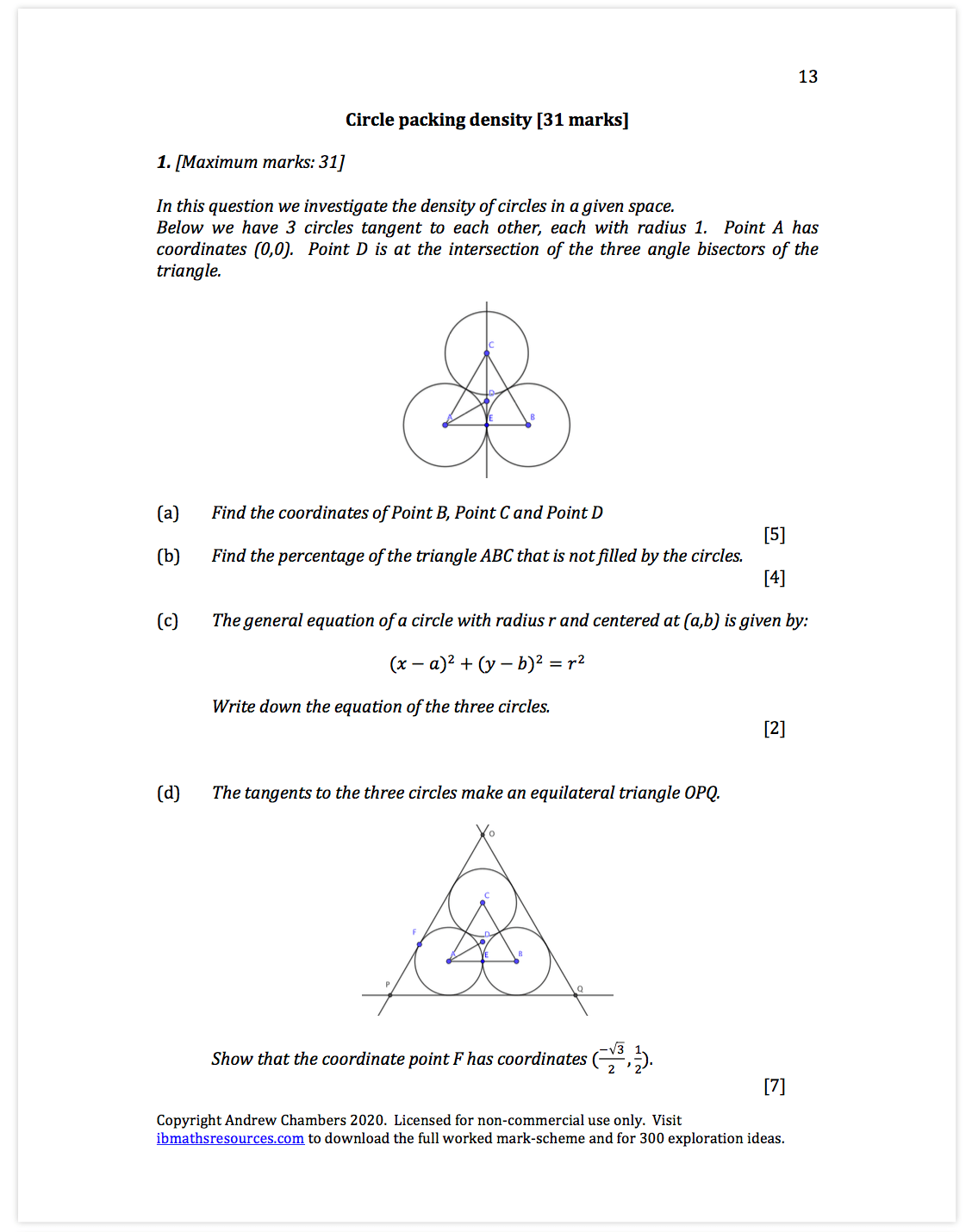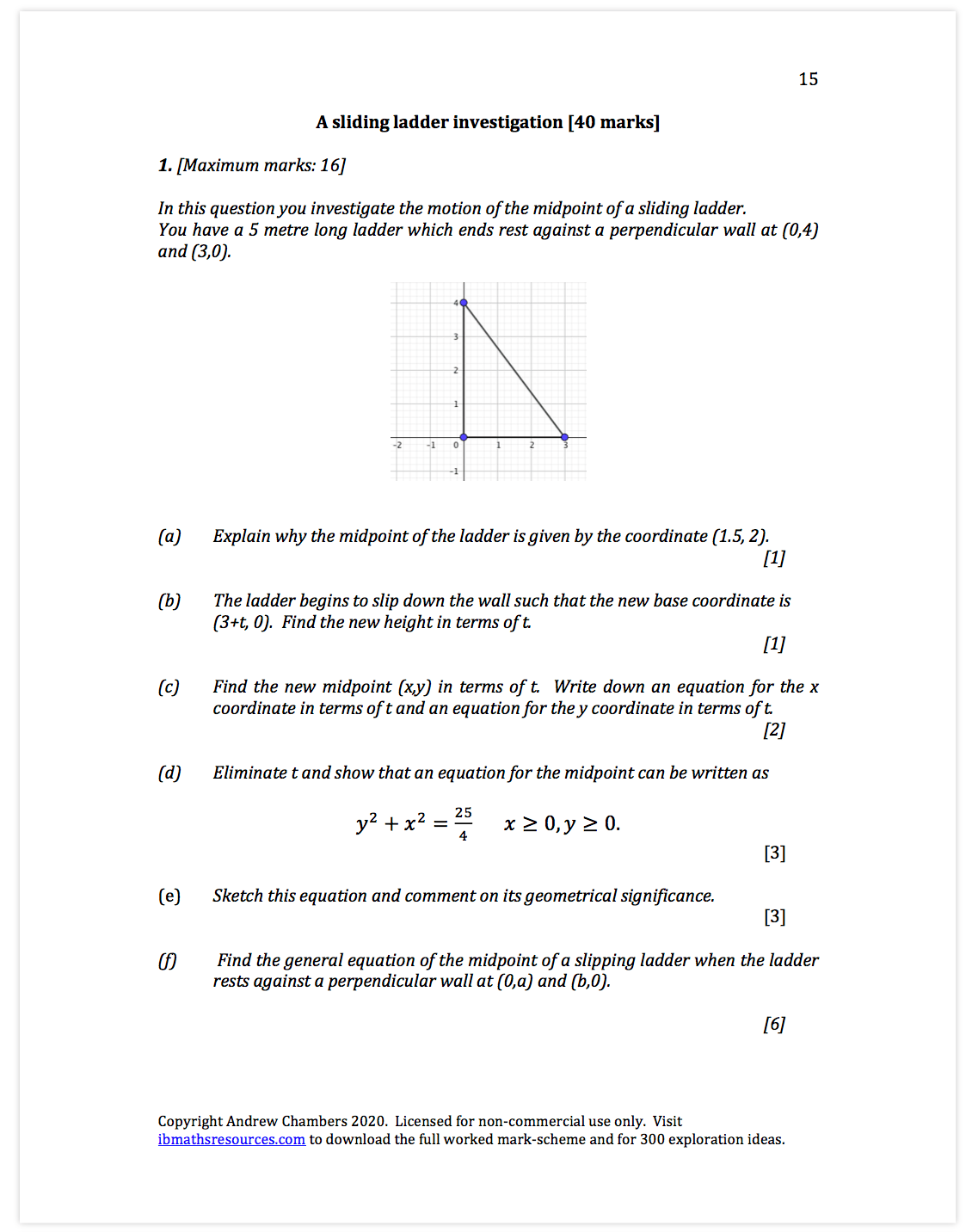# Paper 3 Free Preview

Topics explored:

I have made 26 investigation papers to help with both Paper 3 HL and also the exploration.  Below are 6 of them:

Paper 1: Rotating curves:

Students explore the use of parametric and Cartesian equations to rotate a curve around the origin.  The mathematics used here is trigonometry (identities and triangles), functions and transformations.Paper 2: Who killed Mr. Potato?

Students explore Newton’s Law of Cooling to predict when a potato was removed from an oven.  The mathematics used here is logs laws, linear regression and solving differential equations.Paper 3:  Graphically understanding complex roots

Students explore graphical methods for finding complex roots of quadratics and cubics.  The mathematics used here is complex numbers (finding roots), the sum and product of roots, factor and remainder theorems, equations of tangents.Paper 4: Avoiding a magical barrier

Students explore a scenario that requires them to solve increasingly difficult optimization problems to find the best way of avoiding a barrier.  The mathematics used here is creating equations, optimization and probability.Paper 5 : Circle packing density

Students explore different methods of filling a space with circles to find different circle packing densities.  The mathematics used here is trigonometry and using equations of tangents to find intersection points.Paper 6:  A sliding ladder investigation

Students find the general equation of the midpoint of a slipping ladder and calculate the length of the astroid formed.  The mathematics used here is trigonometry and differentiation (including implicit differentiation).  Students are introduced to the ideas of parametric equations.